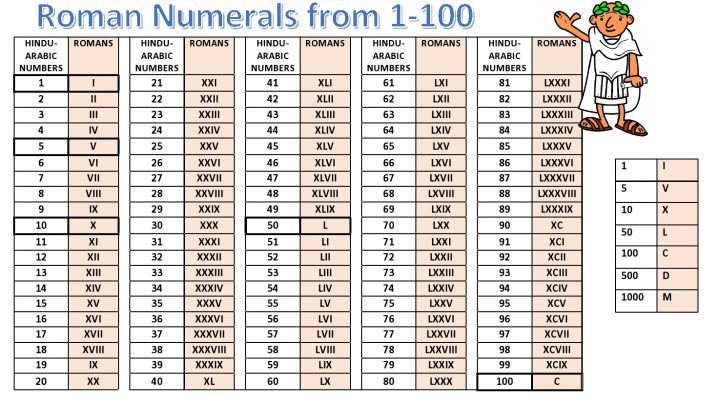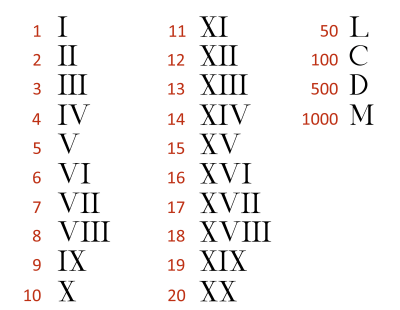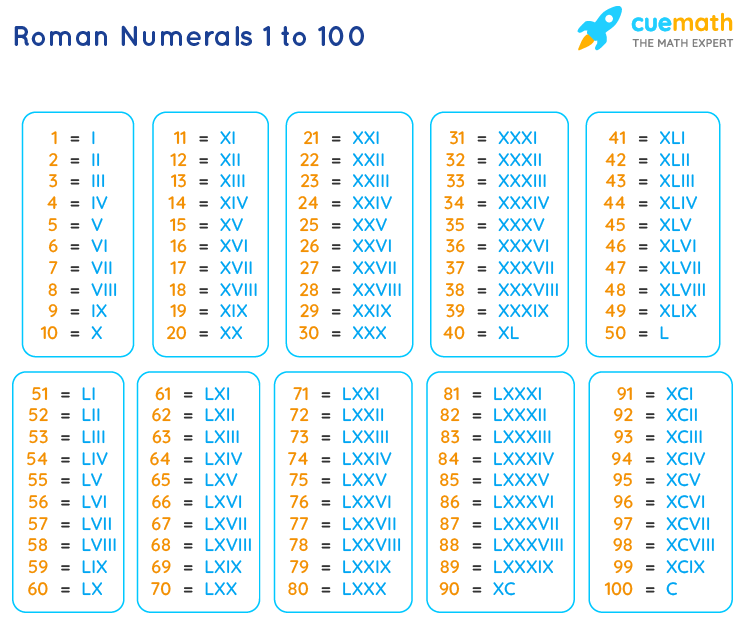# Roman numerals- how to use in MS word, calculator, numbersSo, there are various ways in which you can express numbers. In the modern-day, we mostly use the positional decimal system. We apply it for both just denoting numbers or for mathematical calculations of every kind. So, it has 0 in the middle of the set and there is a negative and positive infinity on both of its sides. Moreover, there are nine digits, excluding 0, that can represent every number in perfect combinations. Now, this is what partially brings us to the Roman numerals. So, we are not unfamiliar with Roman numerals. It is true we never use them for doing sums but they still find a lot of other uses. Roman numerals are extremely popular as size markers in the clothing industry. Moreover, we also often use them for denoting a rank or numbers when we are taking down something. So, they are not obsolete.However, Roman numerals are very different from the decimal number system. You can never use them for numericals because the Romans who invented it did not know about arithmetic. But, most importantly, you cannot because Romans did not know about the use of 0. So, the Roman numerals system has got no 0. However, the pattern is to an extent similar to the decimal system. That is, both are repetitive. However, the pattern that Roman numerals follow is difficult and tedious. That is also a reason why you cannot use them during calculations. We will see more of it in the following sections.

### Roman numerals history

So like every other invention, the Roman numerals too have got a bit of history. Now, in ancient times- times when, say the Iliad or the Odyssey is circulated, there was a constant tension of power between the Romans and the Greeks. These two countries emerged as the then superpowers. So, they had the best rulers, best artists, best armies, and best inventions. However, to compete with each other, they often ended up having systems that were very similar. For example, they both worshiped the same gods and goddesses just with different names.

Now, the ancient Romans developed the system of Roman numerals around 500 BC. So, during this time, the Romans conquered most of the world that they knew. As a result, the Roman numerals flourished throughout Europe. Therefore, this system remained the primary mode of number representation there for centuries. In fact, kings and queens all over Europe used Roman numerals when they repeated a name. They still do, like Elizabeth II. Moreover, England had around 8 Henry, all with a suffix of this numeral system. However, around 1300 AD Roman the more effective Hindu-Arabic system came. So it virtually replaced the Roman numerals all over Europe. Hence, we use it even today.

Now, the symbols that the Romans chose for representation have various sources. So, some even have their homes in the Greek numeral system. However, the Greeks were extremely interested in pure mathematics, such as number theory, geometric proofs, and other abstract ideas. But, the Romans were not. On the other hand, they preferred utilitarian mathematics. So, they primarily used it to figure out personal and government accounts, keep military records and help in the construction of aqueducts and buildings. However, the Roman numeral system allowed for simple addition and subtraction.### Roman numerals how to type in Microsoft word

The origin of I to represent one is straightforward. So, the straight line shows counting on one’s hand, where one finger, which resembles I, equals one of whatever one has to count. They chose the V to represent five. So, this is because supposing you are counting five items with the help of your fingers. Therefore, when you have stretched all five fingers, there is a V between your thumb and forefinger. So, there are various explanations like these- not all of which we really need to know sitting today.Hence, before we learn how to type them in MS- Word, let us take a note of how we should write them in general.

### Roman numerals symbols

So, initially, the Romans adopted the Greek letter X, or chi, to represent 50. However, when historians studied monument transcriptions, they realized that L replaced X as 50. So, now we know that X came to represent 10. However, we do not exactly know how X came to represent 10. So, there are several theories regarding this.

One of those suggests that X came from one V, or five, placed on top of another, upside-down V. Hence, the two Vs formed an X. Another theory took into account the process of counting to 10. So, Romans did this by making ten vertical marks and then crossing them out with an X in order to easily count groups of ten.

If you realize, we see such a thing in stats a lot. This is almost the same way in which Americans keep tallies by groups of five. So, they have four vertical marks that they cross through with a fifth diagonal mark. Eventually, the Romans adopted just X to be the numeral for 10. Moreover, they used the symbol C to represent 100. This is because it is the first letter of the Latin word for one hundred, centum. Similarly, they used M for denoting 1000. So, this is because the Latin word for one thousand is mille.

So, for every unit till 5, you must add on an I. From 5, for every unit, you should add an I with a V. Hence, this is the pattern that continues with L and M too.### Ways to write Roman numerals in MS- Word.

MS- Word is a very accessible application. So, we use it widely. Therefore, it is important that we know how to type Roman numerals in it. The process is pretty easy. However, there are three ways by which you can accomplish this. Let us see all three and then pick up the one that you think is the easiest for you.

#### 1.    By inserting Roman Numerals with Alphabets

Now, we all realize that Roman numerals look very much like English alphabets. It is true that they are not exactly the same. However, I as 1, or X as 10 is quite understandable. So, this is the simplest approach that you can undertake while writing Roman numerals in Word. Just replace them with the English alphabets that look similar. So, we can actually use capital letters like I, V, O, L, C, D, and M to describe the Roman numeral system.

#### 2.    By inserting Roman Numerals by Typing in Unicode

So, this might not be the method that you are most familiar with. Moreover, it is also a bit long and hence, consumes time. However, if you need an exact representation of the Roman numerals, this is important. So, there are 2 steps that you need to follow to get this.

Step 1. You have to just type in the Unicode in a Word document without the ‘U+’ on its front. So next, tap and hold the “Alt” key after typing.

Step 2. While holding the “Alt” key, press “X” to change it in the Roman numerals.

#### 3.    By inserting Roman Numerals with Number Format

However, you might be the one who always uses Roman numerals. So, you might not want to do these substitutes all the time. Therefore, Word has an option to help you. So, you can just set the number format to Roman numerals. And there, you have it.### Roman Numerals to Hindu Arabic

The Hindu-Arabic number system is the one that we use most commonly. So, this is the one that has 0 and we need it for mathematical calculations. However, under various circumstances, we might need to convert the Hindu-Arabic numerals to the Roman numerals and vice-versa. So, as we have already seen the way Roman numerals take up the symbols, let us go through an example. Suppose, you have to convert XLIV.

Now, we know X is 10, I is 1, V is 5, and L is 50. So, these are the general rules. However, that is not exactly how it happens. We do not write IIII for 4, instead, we write IV for 4. So, if you realize that is V- I, so the I comes before V. Therefore, IV is actually 4 here. Now, L too here does not mean 50. So, in the same way, we do not write XXXX for writing 40. Hence, XL means 40. This again comes from the idea that it is 10 or X less than 50 or L. So, as you can guess the number here is 44. Now, 44 is really a small number. It just has 2 digits in it. And yet, converting it manually is not a very fast process.

So, there are high chances you might forget somewhere what symbol means what or what number uses what combination. This is because unlike in the decimal system, the Roman numerals do not have fixed representation in a pattern. So, the best idea is to use a converter or a Roman numerals calculator. Nowadays you find everything on the internet. So, there are various Roman numerals calculators online as well that you can use.### Roman numerals calculator online

So, what we are talking about here is more of a converter than a calculator. To understand the process, we are using the Roman numeral converter from Calculator Soup. Click on the word “Calculator Soup” and it will guide you directly to the parent website where you can find the calculator.

So on opening, you directly see the calculator on the left-hand side of the window. Now let us follow the steps.

### Step 1 to use a Roman numerals calculator

On the top of the calculator, there is a panel. It is written that you can convert roman numerals to numbers and numbers to roman numerals. So, right below that, you have a box that looks like a search engine. Hence, here you have to type your Arabic number or the Roman numeral. So, the converter works both ways. For example, let us consider we type LIII.

### Step 2 to use a Roman numerals calculator

So once you have typed, click the calculate button. It will show “working” and within a couple of seconds, you will find your answer in the panel below. The calculator does not just give you the answer. So, it also shows you the process in which the conversion has taken place. This helps you to understand better.

### Step 3 to use a Roman numerals calculator

So now, check the next panel. At first, you have the answer. So, in this case, you will find LIII = 53. There is a panel below. So, in this panel, you find a table that shows the division. Hence, on one side of the table, you have the roman numerals that are L and III. On the other side, you have the corresponding Arabic numbers that are 50 and 3. So, the calculator adds them up and gives you the final result- that is 53.

So now, you can tap the “clear” button on the first panel to feed in a new set of data.

Therefore, when you have a calculator you do not need to use the old methods. There is no need for a Roman numerals chart from which you can constantly verify if you are doing it right. However, for better understanding, we are giving the conversions for the first few numbers.### Roman numerals calculator 1- 50

1- I

2- II

3- III

4- IV

5- V

6- VI

7- VII

8- VIII

9- IX

10- X

11- XI

12- XII

13- XIII

14- XIV

15- XV

16- XVI

17- XVII

18- XVIII

19- XIX

20- XX

21- XXI

22- XXII

23- XXIII

24- XXIV

25- XXV

26- XXVI

27- XXVII

28- XXVIII

29- XXIX

30- XXX

31- XXXI

32- XXXII

33- XXXIII

34- XXXIV

35- XXXV

36- XXXVI

37- XXXVII

38- XXXVIII

39- XXXIX

40- XL

41- XLI

42- XLII

43- XLIII

44- XLIV

45- XLV

46- XLVI

47- XLVII

48- XLVIII

49- XLIX

50- L### Roman numerals HTML entities

So, for all roman numerals, there is a corresponding HTML code, HTML entity, CSS code, hex code, and Unicode when you want to convert them. Each of the HTML codes, HTML entities, CSS codes, hex codes, and Unicode are different for different Roman numerals. Moreover, they also differ from capitalization. For example,-

For roman numeral one or I, the HTML code is &#8544, the CSS code is \2160, the hex code is &#x2160 and the Unicode is U+02160.

On the other hand, for a small roman numeral one i, the HTML code is &#860, the CSS code is \2170, the hex code is &#x2170 and the Unicode is U+02170.

### Roman numerals calculator python

So, as you can guess, all these calculators work on programming. Therefore, let us take a look at how you can use a python class to convert a roman numeral into an integer.

class py_solution:

def roman_to_int(self, s):

rom_val = {‘I’: 1, ‘V’: 5, ‘X’: 10, ‘L’: 50, ‘C’: 100, ‘D’: 500, ‘M’: 1000}

int_val = 0

for i in range(len(s)):

if i > 0 and rom_val[s[i]] > rom_val[s[i – 1]]:

int_val += rom_val[s[i]] – 2 * rom_val[s[i – 1]]

else:

int_val += rom_val[s[i]]

return int_val

print(py_solution().roman_to_int(‘MMMCMLXXXVI’))

print(py_solution().roman_to_int(‘MMMM’))

print(py_solution().roman_to_int(‘C’))

So, this is how the sample code looks technically. Therefore, the sample output that you will be getting is 3986, 4000, 100.#### Roman Numerals FAQs

##### What are the rules for Roman numerals?

Ans. So, there are a few rules that you need to follow while writing Roman numerals. They are-

• You can repeat the letters I, X, C thrice in succession.
• If you see a lower value digit next to the left of a higher value digit, it means you subtract it.
• So, If you find a lower value digit is next to the right of a higher value digit, you know its addition.
• You can use only I, X, and C as subtractive numerals.
##### Why do Roman numerals have letters?

Ans. So, as we have already seen, Roman numerals were not meant to do sums. So, they had very basic origins in ancient Rome. They came from the shape of fingers or things they regularly used to make counting look easy and simple. Therefore, it was important that they could use the numerals easily during communication. Moreover, having letters made it easy during the trade. And, the idea of digits came a lot later with the Arabic system.

##### How many times can Roman numerals be repeated?

Ans. So, you can repeat I, X, C, and M a maximum of three times. However, you can use the others that are V, L, and D only once.##### Are there any patterns in Roman numerals?

Ans. Yes, there are patterns in Roman numerals. However, it is not purely additive by nature, so the system might get a little unpredictable as you deal with the higher numbers. For example, we write 4 as IV and not IIII. So, the patterns depend on the rules for writing Roman numerals that you can find right above.

##### Is Roman numeral a positional number system?

Ans. No. Therefore, Roman numerals form a non-positional number system. It is because they use different symbols to represent different numbers, unlike the decimal system.

##### Why is there no zero in Roman numerals?

Ans. This is because the Romans did not use Roman numerals for arithmetic. So, it was just every day counting and denoting objects. Moreover, the idea of zero came much later.

##### What does M stand for in Roman numerals?

Ans. So, in Roman numerals, we use an M to mean 1000.

##### What is unique about Roman numerals?

Ans. Well, the most unique thing about Roman numerals is also its greatest drawback. Therefore, it is the lack of zero in the number system. It prevents any higher use of the system.

##### How is 5000 in Roman numerals?

Ans. So, you write 5000 in Roman numerals as V̅. This means it is actually a roman V with a vinculum or bar on top of it.##### How high do Roman numerals go?

Ans. So, this is yet another drawback of the system. Roman numerals do not go till infinity like the decimal number system because they were not designed for finer mathematical calculations. As you might have noticed, the highest Roman numerals can be assigned is an M which is 1000. So, the highest number that you can write with Roman numerals is MMMCMXCIX, or 3,999.